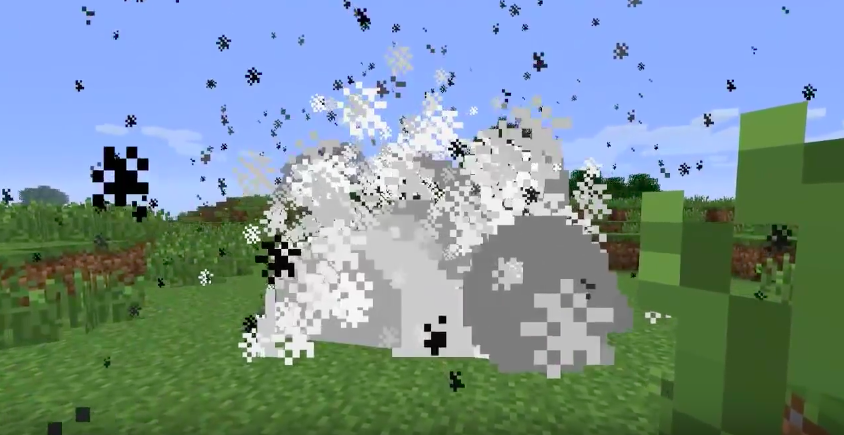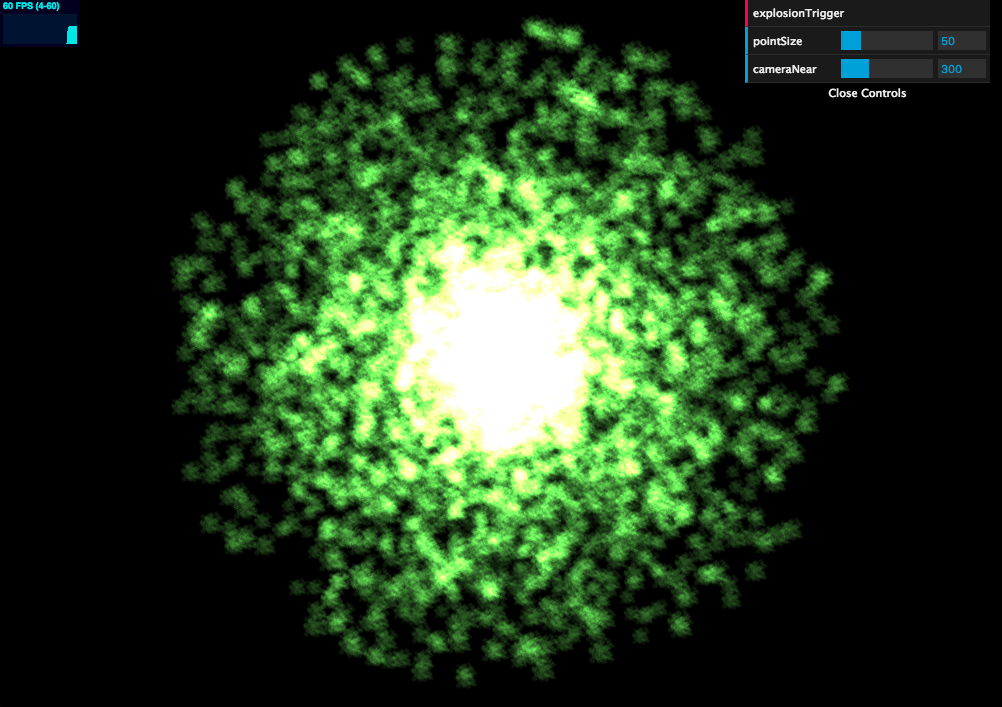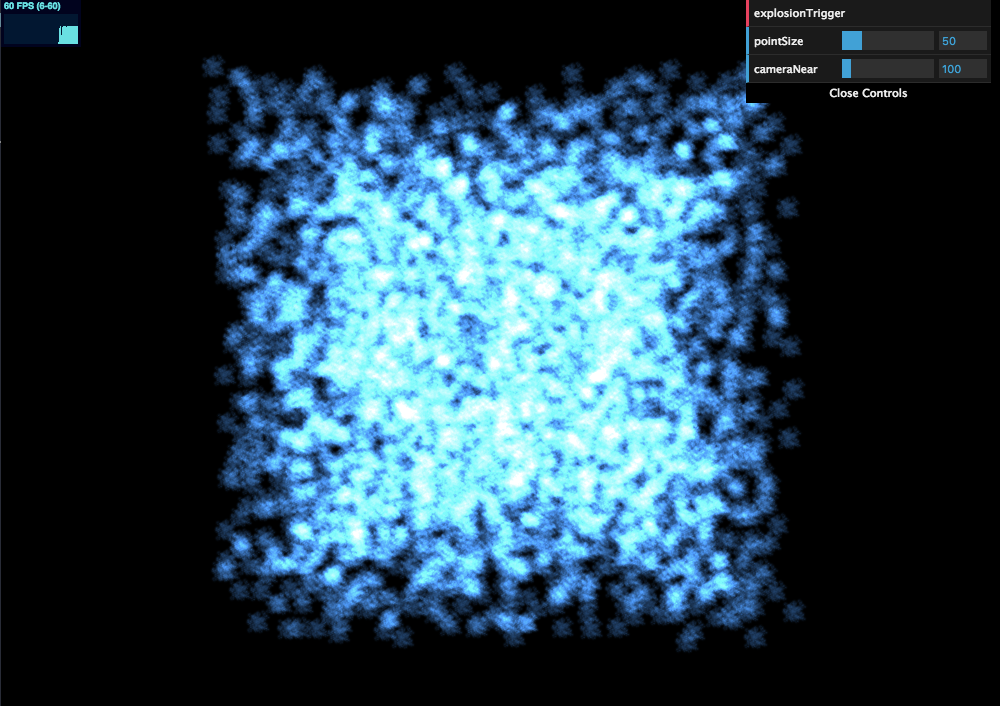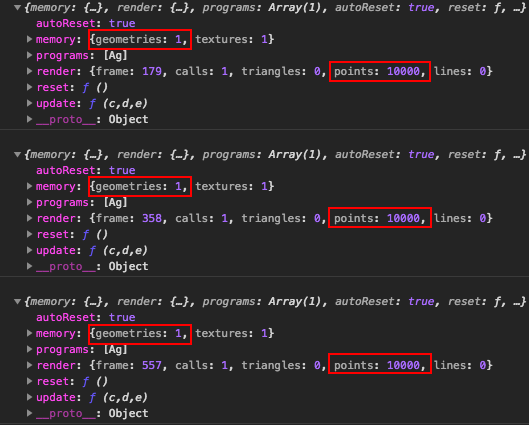#2019 iT 邦幫忙鐵人賽DAY 18
3
Modern Web

## 用 Three.js 來當個創世神 (17)：粒子系統 - 爆炸Photo by Erwan Hesry on Unsplash

## 爆炸效果怎麼做1. 先在某一個相同的爆炸源點建立一群粒子頂點
2. 每個粒子有各自的運動方向（可視為是一個三維向量）
3. 爆炸被觸發後，各粒子依循自己的爆炸方向位置一直疊加上去，直到超出視野則會看不到，會有向四面八方擴散的效果。

## 爆炸實作### 1. 建立粒子系統

``````// 變數宣告
const pointCount = 10000 // 粒子總數
const movementSpeed = 10 // 移速種子
let explosion // 爆炸物件
let size = 50 // 粒子尺寸
``````
``````// 爆炸類別
class Explosion {
constructor(x, y) {

// 幾何體
const geometry = new THREE.Geometry()

// 材質
this.material = new THREE.PointsMaterial({
size: size,
color: new THREE.Color(Math.random() * 0xffffff), // 顏色隨機
map: smokeTexture,
depthTest: false,
transparent: true,
opacity: 0.7
})

this.pCount = pointCount
this.movementSpeed = movementSpeed
this.dirs = []

// 建立粒子系統所需頂點
for (let i = 0; i < this.pCount; i++) {
const vertex = new THREE.Vector3(x, y, 0) // 每個頂點起點都在爆炸起源點
geometry.vertices.push(vertex)
}

let points = new THREE.Points(geometry, this.material)

this.object = points

}
}
``````

### 2. 準備爆炸方向屬性

``````class Explosion {
constructor(x, y) {
...
for (let i = 0; i < this.pCount; i++) {
...
const r = this.movementSpeed * THREE.Math.randFloat(0, 1)
// 噴射方向隨機 -> 不規則球體
const theta = Math.random() * Math.PI * 2
const phi = Math.random() * Math.PI
this.dirs.push({
x: r * Math.sin(phi) * Math.cos(theta),
y: r * Math.sin(phi) * Math.sin(theta),
z: r * Math.cos(phi)
})
}
}
}
``````### 3. 爆炸特效動畫

``````class Explosion {
...
update() {
let p = this.pCount
const d = this.dirs
while (p--) {
let particle = this.object.geometry.vertices[p]
particle.x += d[p].x
particle.y += d[p].y
particle.z += d[p].z
}
this.object.geometry.verticesNeedUpdate = true
}
}
``````
``````function render() {
if (explosion) {
explosion.update()
}
...
requestAnimationFrame(render)
renderer.render(scene, camera)
}
``````

## 釋放記憶體與驗證

``````function render() {
var geometry = new THREE.SphereBufferGeometry(...);
var material = new THREE.MeshBasicMaterial(...);
var mesh = new THREE.Mesh( geometry, material );
renderer.render( scene, camera );
scene.remove( mesh );
// clean up
geometry.dispose();
material.dispose();
texture.dispose();
}
``````

``````class Explosion {
...
destroy() {
this.object.geometry.dispose()
scene.remove(this.object)
// console.log(renderer.info) // 驗證
this.dirs.length = 0
}
}
``````

``````this.explosionTrigger = function() {
if (explosion) {
explosion.destroy()
}
explosion = new Explosion(0, 0)
}
``````

### 沒做 destroy 的版本：### 有做 destroy 的版本：